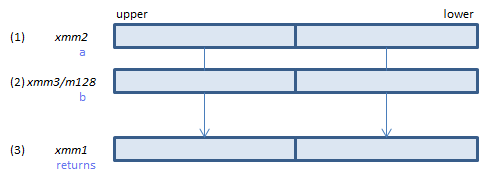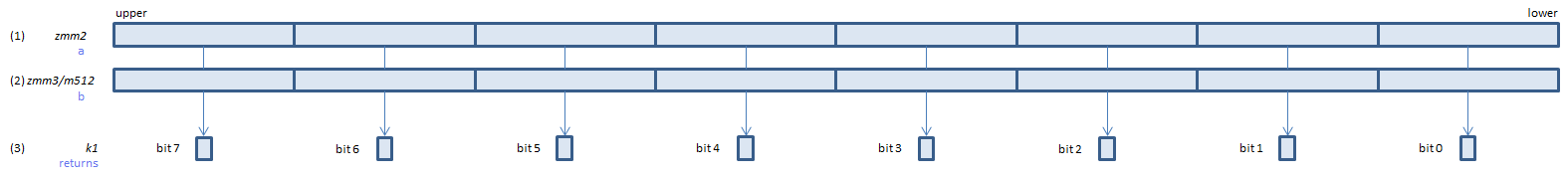﻿ vcmpunord_spd

## VCMPUNORD_SPD - CoMPare UNORDered Signal Packed Double

VCMPUNORD_SPD xmm1, xmm2, xmm3/m128    (V1
__m128d _mm_cmp_pd(__m128d a, __m128d b, _CMP_UNORD_S)For each double, if either or both of (1)(2) is NaN, set 1, else set 0, to all bits of the corresponding double of (3).
VCMPUNORD_SPD ymm1, ymm2, ymm3/m256    (V1
__m256d _mm256_cmp_pd(__m256d a, __m256d b, _CMP_UNORD_S)For each double, if either or both of (1)(2) is NaN, set 1, else set 0, to all bits of the corresponding double of (3).
VCMPUNORD_SPD k1{k2}, xmm2, xmm3/m128/m64bcst    (V5+VLFor each double, if either or both of (1)(2) is NaN, set 1, else set 0, to the corresponding bit of (3).
If k2 bit is 0, the comparison is not done and the corresponding bit of (3) is set to zero. Upper bits of (3) are zero cleared.
VCMPUNORD_SPD k1{k2}, ymm2, ymm3/m256/m64bcst    (V5+VLFor each double, if either or both of (1)(2) is NaN, set 1, else set 0, to the corresponding bit of (3).
If k2 bit is 0, the comparison is not done and the corresponding bit of (3) is set to zero. Upper bits of (3) are zero cleared.
VCMPUNORD_SPD k1{k2}, zmm2, zmm3/m512/m64bcst{sae}    (V5